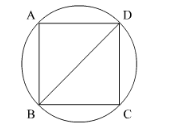# If a square is inscribed in a circle,Question:

If a square is inscribed in a circle, what is the ratio of the areas of the circle and the square?

Solution:

We have the following situationLet BD be the diameter and diagonal of the circle and the square respectively.

We know that area of the circle $=\pi r^{2}$

Area of the square $=\operatorname{side}^{2}$

As we know that diagonal of the square is the diameter of the square.

Diagonal $=2 r$

Side of the square $=\frac{\text { diagonal }}{\sqrt{2}}$......(1)

Substituting diagonal $=2 r$ in equation (1) we get,

Side of the square $=\frac{2 r}{\sqrt{2}}$

Now we will find the ratio of the areas of circle and square.

$\frac{\text { Area of circle }}{\text { Area of square }}=\frac{\pi r^{2}}{\left(\frac{2 r}{\sqrt{2}}\right)^{2}}$

Now we will simplify the above equation as below,

$\frac{\text { Area of circle }}{\text { Area of square }}=\frac{\pi r^{2}}{\frac{4 r^{2}}{2}}$

$\frac{\text { Area of circle }}{\text { Area of square }}=\pi r^{2} \times \frac{2}{4 r^{2}}$

Hence, $\frac{\text { Area of circle }}{\text { Area of square }}=\frac{\pi}{2}$

Therefore, ratio of areas of circle and square is $\pi: 2$.